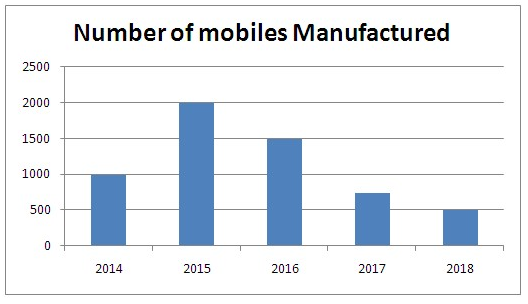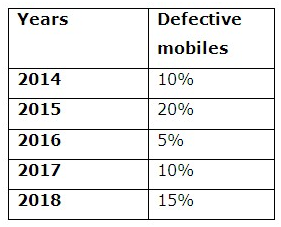# SBI PO Prelims Quantitative Aptitude (Day-04)

Dear Aspirants, Our IBPS Guide team is providing new series of Quantitative Aptitude Questions for SBI PO 2020 so the aspirants can practice it on a daily basis. These questions are framed by our skilled experts after understanding your needs thoroughly. Aspirants can practice these new series questions daily to familiarize with the exact exam pattern and make your preparation effective.

Start Quiz

Data Interpretation

Directions (01 – 06): Study the following information carefully and answer the given questions

The given bar graph shows the number of mobiles manufactured in 5 different years.The given table shows the percentage of mobile is defective in 5 different years.1)  If the number of non defective mobiles sold in 2015, 2017 and 2018 is 90%, 80% and 60% respectively, find the average number mobiles unsold in 2015, 2018 and 2017 together?

A) 145

B) 155

C) 165

D) 135

E) None of these

2)  The number of defective mobiles in 2014 is approximately what percent of the number of non defective mobiles in 2016?

A) 7%

B) 9%

C) 11%

D) 3%

E) 15%

3)  If the number of non defective mobiles sold in 2015 is 75% and the selling price of a mobile is Rs.6000, then find the total revenue in 2015?

A) Rs.8200000

B) Rs.7200000

C) Rs.8400000

D) Rs.7400000

E) None of these

4) In next year 2019, the number of manufacturing is increased by 20% compared to previous year and the defective mobile is 15% and the unsold mobile is 20%, then find the unsold mobiles in 2019?

A) 102

B) 107

C) 108

D) 103

E) None of these

5) Find the average number of defective mobiles in all the five years together?

A) 135

B) 125

C) 145

D) 155

E) None of these

6) The number of non defective mobile in 2017 is what percent more than that of the number of mobile manufactured in 2018?

A) 35%

B) 25%

C) 15%

D) 5%

E) None of these

Data Sufficiency

Directions (07 – 10): The following questions are accompanied by two statements I and II. You have to determine which statement is/are sufficient to answer the question.

7) What is the rate of interest?

Statement I: If the difference between the SI and CI for three years is Rs.1840.

Statement II: Ram invests Rs.5000 for 5 years and after 5 years he received Rs.3600.

A) Only I

B) Only II

C) Either I or II

D) Both I and II

E) Neither I nor II

8) Find the value of x

Statement I: A and B started a business with investment in the ratio of 3: 2 and after x months A withdrew half of his initial investment.

Statement II: A and B started the business and at the end one year they share the profit in the ratio of 7:8

A) Only I

B) Only II

C) Either I or II

D) Both I and II

E) Neither I nor II

9)  Find the radius of the circle?

Statement I: If the ratio of radius of circle to cylinder is 2: 1 and the volume of the cylinder is 2634 cm3.

Statement II: If the radius of the circle is equal to the radius of the cone and the height and length of the cone are 24 cm and 25 cm respectively.

A) Only I

B) Only II

C) Either I or II

D) Both I and II

E) Neither I nor II

10)  What of the ratio of speed of train A to speed of train B?

Statement I: If train A crosses the 200 m long train B running in opposite direction in 36 seconds and the train A also crosses the pole in 18 seconds.

Statement II: Train B crosses the 180 m long tunnel in 54 seconds and also crosses the train A running in same direction in 54 seconds.

A) Only I

B) Only II

C) Either I or II

D) Both I and II

E) Neither I nor II

Directions (1-5) :

Non defective mobiles in 2015 = 80/100 * 2000 = 1600

Unsold mobile in 2015 = 10/100 * 1600 = 160

Non defective mobiles in 2017 = 750 * 90/100 = 675

Unsold mobile in 2017 = 20/100 * 675 = 135

Non defective mobiles in 2018 = 500 * 85/100 = 425

Unsold mobile in 2018 = 425 * 40/100 = 170

Average = (160 + 135 + 170)/3 = 155

Defective mobile in 2014 = 1000 * 10/100 = 100

Non defective mobile in 2016 = 1500 * 95/100 = 1425

Required percentage = 100/1425 * 100 = 7%

Non defective mobiles in 2015 = 80/100 * 2000 = 1600

Sold mobile = 1600 * 75/100 = 1200

Revenue = 1200 * 6000 = 7200000

Manufacturing = 500 * 120/100 = 600

Non defective = 85/100 * 600 = 510

Unsold = 510 * 20/100 = 102

Defective in 2014 = 1000 * 10/100 = 100

Defective in 2015 = 2000 * 20/100 = 400

Defective in 2016 = 1500 * 5/100 = 75

Defective in 2017 = 750 * 10/100 = 75

Defective in 2018 = 500 * 15/100 = 75

Average = (100 + 400 + 75 + 75 + 75)/5 = 145

Non defective mobiles in 2017 = 750 * 90/100 = 675

Number of mobile manufactured = 500

Required percentage = (675 – 500)/500 * 100 = 35%

Directions (7-10) :

From statement I,

SI and CI difference for 3 years = (PR2/1003) * (R +300)

Difference = 1840

Sum is not given.

So, Statement I alone is not sufficient to answer the question.

From Statement II,

Ram invests his amount in Simple interest or compound interest is not known.

So, Statement II alone is not sufficient to the answer the question.

Hence, both the statements are not necessary to answer the given question.

From statement I,

Investment ratio of A to B = 3: 2

A withdrew his amount after some period, but we don’t know the exact time period.

So, Statement I alone is not sufficient to the answer the question.

From Statement II,

Profit ratio of A to B =7: 8

So, Statement II alone is not sufficient to the answer the question.

From I and II,

(3 * x + (1.5 * (12 – x)): (2 * 12) = 7: 8

(3x + 18 – 1.5x) / 24 = 7/8

(1.5x + 18)/24 = 7/8

= > 1.5x + 18 = 21

= > 1.5x = 3

= > x = 2

Hence, both the statements are necessary to answer the given question.

From Statement I,

Radius of Circle to cylinder = 2: 1

Volume of the cylinder = 2634 cm3

So, Statement I alone is not sufficient to the answer the question.

From Statement II,

Slant height (l) = √(r2 + h2)

25 = √(24 * 24 + r * r)

625 = 576 + r * r

Radius of the cone = 7 cm

Radius of the circle = 7 cm

So, Statement II alone is sufficient to the answer the question.

Hence, statement II alone is sufficient to answer the given question.

From Statement I,

Length of train A = x

Speed of train A = a

Speed of train B = b

(x + 200) = (a + b) * 5/18 * 36

(x + 200) = (a + b) * 10 —- (1)

= > x = a * 5/18 * 18

= > x = 5a —- (2)

Substitute the equation (2) in (1),

(x + 200) = (x/5 + b) * 10

So, Statement I alone is not sufficient to the answer the question.

From Statement II,

Length of train A = x

Length of train B = y

Speed of train B = b

Speed of train A = a

(y + 180) = b * 5/18 * 54

(y + 180) = 15b —- (1)

(x + y) = (a – b) * 5/18 * 54

(x + y) = (a – b) * 15 —- (2)

So, Statement II alone is not sufficient to the answer the question.

 Check Here to View SBI PO Prelims 2020 Quantitative Aptitude Questions Day – 03 Day – 02 Day – 01 Click Here for SBI Clerk 2020 – Detailed Exam Notification